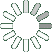Puzzle Command
Daily Puzzle
Number Logic #13
January 25, 2015Find a 6 digit number where:

The 1st digit is 20 divided by 4.
The 2nd digit is 100 divided by 20.
The 3rd digit is not equal to 5.
The 4th digit is 3 times 3 times 64 divided by 8 divided by 8.
The 5th digit is the sum of the 3rd and 4th digit.
The 6th digit is the sum of the 1st and 3rd digit.

Puzzle Notes
6 digit numbers range from 100,000 to 999,999.
Enter in your solution below and click submit.